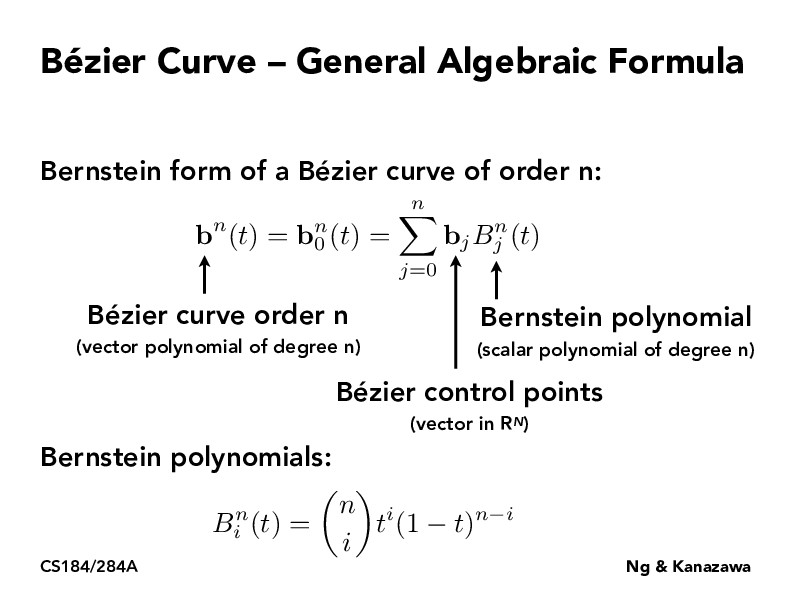Lecture 7: Splines and Bezier Curves (70)aagarwal1999

For the math nerds out there, it turns out the Berstein Polynomials are a key point in a proof that any real valued function can be approximated by a polynomial (Weirstrass Approximation Theorem).

You can find a proof listing here: https://en.wikipedia.org/wiki/Bernstein_polynomial#Approximating_continuous_functions

You must be enrolled in the course to comment